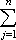#Interactive Real Analysis

Next | Previous | Glossary | Map

## 7.1. Riemann Integral

### Examples 7.1.9(b):

Is the function f(x) = x2 Riemann integrable on the interval [0,1] ? If so, find the value of the Riemann integral. Do the same for the interval [-1, 1].
First let's experiment with our "Integrator" applet. To "show" that f(x) = x2 is integrable we need to take partitions with more and more points, compute the upper and lower sum, and hope that the numeric answers will get closer and closer to one common value. If that's the case, our guess is that this limit is the integral of f over the indicated interval.

But of course that's no proof. As it turns out, to prove that this simple function is integrable will be difficult, because we do not have a simple condition at our disposal that could tell us quickly whether this, or any other function, is integrable. That's the bad news; the good news will be that we should be able to generalize the proof for this particular example to a wider set of functions.

Anyhow, here we go. First we should note that in the definition of upper and lower integral it is not necessary to take the sup and inf over all partitions. After all, if P is a partition and P' is a refinement of P then L(f, P')L(f, P) and U(f, P')U(f, P). Therefore partitions with large norm don't contribute to the sup or inf and it is enough to compute the upper and lower integral by considering partitions with a small norm only.

Next, take any> 0 and a partition P with |P| </ 2. Then

| U(f, P) - L(f, P)||cj - dj| (xj - xj-1)
where cj is the sup of f over [xj-1, xj] and dj is the inf over that interval.

Since f is increasing over [0, 1] we know that the sup is achieved on the right side of each subinterval, the inf on the left side. Therefore:

| U(f, P) - L(f, P)||cj - dj| (xj - xj-1) =|f(xj) - f(xj-1)| (xj - xj-1)

To estimate this sum, we'll apply the Mean Value Theorem for f(x) = x2:
|f(x) - f(y)||f'(c)| |x - y|
for c between x and y. Since |f'(c)|2 for c in the interval [0, 1] we know that
|f(x) - f(y)|2 |x - y|
But the partition P was chosen with |P| </ 2 so that
|f(xj) - f(xj-1)|2 |xj - xj-1|2/ 2 =But then
| U(f, P) - L(f, P)||f(xj) - f(xj)| (xj - xj-1)(xj - xj-1) =(xn - x0)
=(1 - 0) =because the last sum is telescoping.

Since the partition P was arbitrary but with small norm - which we remarked is sufficient for the upper and lower integral - we know that the upper and lower integral must exist and be equal to one common limit L.

Therefore we know that f is integrable and it remains to find the value L of the integral. But that's easy to compute because now that we know that the function is integrable we can take a suitable partition to find the value of the integral. So, take the following partition:

xj = j/n
for j = 0, 1, 2, ..., n. Then the upper sum computes to
U(f, P) =cj (xj - xj-1) =f(xj) 1/n
=(j/n)2 1/n = 1/n3j 2
= 1/n3 1/6 n (n+1) (2n+1) = 1/6 (n+1) (2n+1) / n2
We know that the upper integral exists and is equal to L. Therefore, the limit as n goes to infinity of the above expression must also converge to L. But then L = 1/3, or in other words:x2 dx = 1/3
where a = 0 and b = 1.

As for the interval [-1, 1], we can first play our applet game to get an idea about the answer, then set out to prove everything just as we did above.

It seems that the applet says that the integral now should evaluate to something close to 2/3. Of course we need some formal proof, but since that would be similar to the above one we'll leave it as an exercise.

The proof is somewhat misleading, because it seems to be based on the fact that f(x) = x2 is differentiable. As a generalization we might conclude that differentiable functions are integrable. That's correct, but another, more general concept can be substituted for differentiability (what might it be?).

Next | Previous | Glossary | Map